# Alcohol from potatoes

In the distillery, 10 hl of alcohol can make from 8 t of potatoes. The rectangular field with dimensions of 600 m and 200 m had a yield of 20 t of potatoes per hectare. How many square meters of area are potatoes grown to obtain one liter of alcohol?

x =  4 m2

### Step-by-step explanation: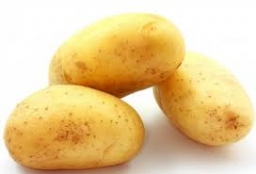Did you find an error or inaccuracy? Feel free to write us. Thank you!Tips to related online calculators
Do you want to convert area units?
Do you know the volume and unit volume, and want to convert volume units?
Do you want to convert mass units?

#### You need to know the following knowledge to solve this word math problem:

We encourage you to watch this tutorial video on this math problem:

## Related math problems and questions:

• SeedsThe field has a rectangular shape with dimensions of 128 m and 350 m. How many kg of seed are needed for sowing if the 1 m2 will consume 25 g of seeds?
• Sunflower Field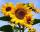The trapezoidal sunflower field is located between two parallel paths which are spaced 230 meters apart. The lengths of the parallel sides of the field are 255 m and 274 m. How many tons of sunflower will come from this field if the hectare yield is 2.25
• Argicultural fieldThe field has a rectangular shape with a width of 180 m and a circumference of 940 m. How many hectares acreage has field?
• Basic formExpressed the ratios of values in the basic form: 0,5 t : 1,2 kg 200 l : 0,15 m3 12 t : 3600 kg 500 kg : 2,5 t 0,9 kg : 500 g 3,6 m : 240 cm 1200 mm : 2,4 m 300 l : 0,3 m3 6 min 30 s : 900 s
• PotatoesWhen planting potatoes there are consumed 3 500 kg of seed per 1 ha. Calculate the weight of the seed needed for fitting a square area with a side length 325 meters.
• HectaresThe rectangular land is 350m long and 200m wide. Calculate the area in hectares.
• The fieldThe player crossed the field diagonally and walked the length of 250 m. Calculate the length of the field, circumference if one side of field 25 meters.
• Rectangular fieldOne dimension of the rectangular field is 56 m greater than second dimension. If each side of the rectangle increases by 10 m, increases the surface field is 1480 m2 . Determine dimencions of the field.
• The hallThe hall had a rectangular ground plan one dimension 20 m longer than the other. After rebuilding the length of the hall declined by 5 m and the width has increased by 10 m. Floor area increased by 300 m2. What were the original dimensions of the hall?
• Rectangular fieldA rectangular field has a diagonal of length 169m. If the length and width are in the ratio 12:5. Find the dimensions of the field, the perimeter of the field and the area of the field.
• The poolThe cube-shaped pool has 140 cubic meters of water. Determine the dimensions of the bottom if the depth of the water is 200 cm and one dimension of the bottom is 3 m greater than the other. What are the dimensions of the pool bottom?
• Wheat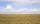The rectangle shaped field with dimensions 1268 m and 745 m harvested last year was 4959 q wheat. (1 q = 1 quintal = 100 kg). During the year, it was necessary to fix the pipe and therefore did kick wide 4 m parallel to the side of the field 745 m, where
• The farmer field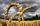The field has a parallelogram shape with dimensions side a = 80 m and height 296 m. Two and two sides is at angle 80°. Calculate acreage of field in hectares.
• Natural fertilizerThe rectangular garden measuring 120m and 60m was fertilized with 16kg of natural fertilizer. Natural fertilizer contains 45% organic matter. How much organic matter falls on 1 m2 of garden?
• The landThe land, which has dimensions of 220 m and 308 m, the owner wants to divide into equally large square plots with the largest possible area. How long will one side of the plot be?
• Fence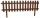How many meters of fencing it is required to purchase if our garden is rectangular measuring 20 m and 180 dm.
• Insulate houseThe property owner wants to insulate his house. The house has these dimensions 12, and 12 m is 15 m high. The windows have 6 with dimensions 170 and 150 cm. Entrance doors are 250 and 170 cm in size. How many square meters of polystyrene does he need?